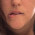Monday, January 18, 2021

Tensorflow / Keras threshold operations break the gradient flow.

There is a way to fix this by using a combination of operations.

def threshold_min_max_value(input_layer,
min_value=0.0,
max_value=1.0):
"""
Thresholds all the values of the a tensor that exceed value to that
max_value, and than are lower than the min_value,

:param input_layer: the input layer
:param min_value: minimum value to threshold to
:param max_value: maximum value to threshold to
:return: threshold-ed input layer
"""

def _threshold(_x):
ge_max_value = K.greater_equal(_x, max_value)
ge_max_value = K.cast_to_floatx(ge_max_value)
lt_max_value = 1.0 - ge_max_value

le_min_value = K.less_equal(_x, min_value)
le_min_value = K.cast_to_floatx(le_min_value)
gt_min_value = 1.0 - le_min_value

tmp0 = keras.layers.Multiply()([
lt_max_value, gt_min_value, _x
])
return tmp0 + (min_value * le_min_value) + (max_value * ge_max_value)

return keras.layers.Lambda(_threshold)(input_layer)1.2.3.4.